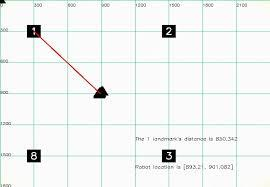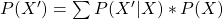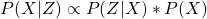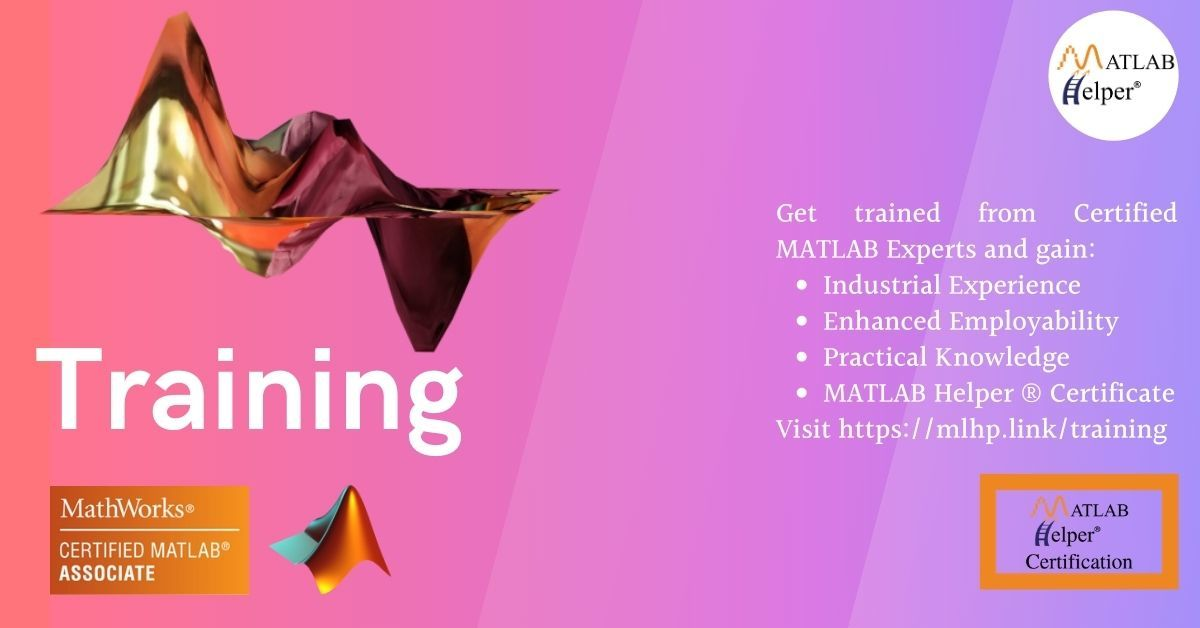# Robot motion simulation & localization with particle filter

April 15, 2022

lang: en_US

Robot Localization is the process by which the location and orientation of the robot within its environment are estimated. The process used for this purpose is the particle filter. This particle filter-based algorithm for robot localization is also known as Monte Carlo Localization. Unlike other filters, such as the Kalman filter and its variants, this algorithm is also designed for arbitrary non-Gaussian and multi-modal distributions. Instead of using a parametric form of distribution, we use the non-parametric samples-based representation where the set of particles represent the hypothesis of where the robot might be. This is a Bayes filter with which the localization is performed.

## Description of the particle filter localization algorithm

Given a floor plan of the cyclic environment of the robot where the robot is located, it has to perform global localization. It has no clue where it is and needs the sensor measurements to find out where it is. The robot uses range sensors to find the distances to its landmarks to obtain a good posterior distribution of its location. The particle filter is used with thousands of particles, giving a discrete random guess of where the robot might be. The state is defined with an X-coordinate, Y-coordinate, and a heading direction which comprises a single guess. So the set of all the thousands of guesses together represents the approximation for the posterior of the robot.

In the beginning, the particles are uniformly spread. The particle filter retains the particles in proportion to how consistent they are with the true measurements and discards the remaining particles. Thus the characteristic of the particle filter is to hone in on the right particles, which makes them survive, and the rest of them don't survive through the algorithm. Therefore the poses of high probability will have more particles cluster around a single location, and so they more accurately represent the robot's posterior beliefRobot Localization Particle Filter

Let's now dive into how this is programmed in MATLAB. The robot is located in a 2-dimensional area, and it can see 4 different landmarks. So the process of making such a robot is straightforward, and all that needs to be done is to call the function 'robot' and assign it to a variable. The set command assigns the robot's pose with x, y, and heading direction in radians, and then the robot is made to move with the move command.The next thing to be done is to generate the measurements using the sense command, which obtains the distances to the four landmarks. The class robot has built-in noise variables such as forward noise, turn noise, and sensor noise, and these are set using a class method. The next method is written for the measurement probability. This takes a measurement and describes how plausible that measurement is. The measured distances to the landmarks comprise the measurement vector of the robot. The particle filter comprises the set of 1000 random guesses as to where the robot might be, which is structured as explained earlier. When the function 'robot' is called and assigned to a particle, the X, Y, and orientation(heading) are initialized randomly, and 1000 such particles are created using an iteration.

The motion of these particles is simulated by assigning the motion parameters for each of these particles and is done similarly to the robot motion. The full recursion of motion update is done to the entire particle set. The next part of the process has the particle hypothesizing on the robot's coordinates and heading direction. Suppose a particle incorrectly predicts the robot pose. The measurement vector is applied to this particle and compared with the actual measured distances to the landmarks. It will be taken as an unlikely particle. The mismatch of the actual measurement with the predicted measurement leads to what is known as importance weights, which measure how important that particle is. So weights are accordingly calculated for each particle, and the probability that the particle is retained in the next sampling step will be proportional to its weight. Then the process of resampling with replacement is done by randomly drawing N new particles independently from this set of particles in direct proportion with the importance weights, i.e., the sampling probability is proportional to the importance weights. After the resampling phase, only the more consistent particles with the sensor measurements survive with a higher probability, and the rest of them would die out.

Thus this creates a cluster of particles around the region of the higher posterior probability. In the code, the importance weights are assigned to the particles depending on the likelihood of the measurement, and the resampling is implemented. The function that implements the calculation of how likely the measurement is, uses a Gaussian, which measures how far the predicted measurements of the particles are from the true measurements of the robot. Then the resampling algorithm is done. The particles with higher probability are drawn more frequently into the next data set than those with lesser probability. This process is done with an algorithm that picks particles in proportion to the fraction of the circumference that it spans by what is known as the resampling wheel. The details of the resampling algorithm by the resampling wheel method are not in the scope of this blog. This implementation is not domain-specific and is generic and easy to program for any application. So this generates a set of co-located particles after the resampling step instead of having a completely random set of particles.

For the first location, the distances to the landmarks are invariant of the orientation. But from the subsequent locations, the orientation will also play a role after the robot moves from the initial position since the measurements to landmarks will be different for different initial orientations. It is assumed the environment is cyclic, i.e., what this means is that the robot will eventually revisit the area that it has already explored.Robot-distance-to-landmarks

## Theory of the particle filter

Two updates are made during the robot's localization: the motion update and the measurements update. In the probabilistic interpretation, a proposal distribution is used to generate samples. The importance weights correct for the differences between the target and proposal distributions. The proposal distribution is the robot's motion model, which is the prediction step. The observation model is used to compute the importance weights, which is the correction step. The simplified version of the probabilistic equations is presented.

• In the motion update, the posterior of the distribution at the next time step is the sum of the transition probabilities of the state to the next time state times the prior probability of the state as,The P(X') is posterior of the state at the next time step is obtained by applying  the motion model and noise model and sampling from the sum over all particles

• In the measurement update, the posterior is computed over the state given the measurement as,• P(X) is the distribution of 1000 particles, and P(Z|X) is the importance weight which is the probability of the measurement given the state. And P(X|Z) represents the state's posterior distribution given the measurements obtained after the resampling step.

The interested reader can refer to 'Probabilistic Robotics' By Sebastian Thrun  for more details on the theory. These are the updates that have been implemented in the MATLAB code taken from MathWorks File Exchange. This code has been adapted from the original application developed by Michael Mathew (2022). In that application, the GUI development environment used MATLAB GUIDE. Only one robot was used for the simulation. That code was adapted from the Python code by Sebastian Thrun. This application has been re-adapted with MATLAB app designer in our modified version. The simulation has been performed for more than one robot using the same original robot.m script.

## MATLAB code for particle filter-based robot localization

Code, GUI, App & Report!

Have you ever thought about how you could estimate the position and orientation of a mobile robot with range sensors on a known map? We can quickly and accurately achieve this using a particle filter to localize the robot. Visualize this simulation in MATLAB and get an intuitive understanding of how the particle filter works; Updated & Tested in MATLAB R2021b with GUIDE and MATLAB R2022a with App Designer

## Results

### GUIDE

This figure shows the output of running the particle filter robot localization. It can be seen that the particle motion follows the robot motion and clusters around the region of the highest probability.Particle Filter simulation

### App Designer

In the modified implementation with App Designer, the results and functionality are similar to the original implementation. The revised version performs the simulation for more than one robot. The robot's trajectory is traced as it moves the specified distance, and a simulation movie is created.## Conclusion

Thus we have seen how a simple and rudimentary simulation of robot localization is done given its map of the environment using the particle filter. The weighted samples of particles are generated within a certain area to represent the locations and orientations of the particles and serve as an approximation to probability density functions.

Did you find some helpful content from our video or article and now looking for its code, model, or application? You can purchase the specific Title, if available, and instantly get the download link.

If you are looking for free help, you can post your comment below & wait for any community member to respond, which is not guaranteed. You can book Expert Help, a paid service, and get assistance in your requirement. If your timeline allows, we recommend you book the Research Assistance plan. If you want to get trained in MATLAB or Simulink, you may join one of our training modules.

If you are ready for the paid service, share your requirement with necessary attachments & inform us about any Service preference along with the timeline. Once evaluated, we will revert to you with more details and the next suggested step.

Education is our future. MATLAB is our feature. Happy MATLABing!

MATLAB Developer at MATLAB Helper,
M.S in Telecommunications and Networking,
M.S in Physics

#### Connect with MATLAB Helper ®

Use Website Chat or WhatsApp at +91-8104622179

Watch our Latest Video

Join a Training Module